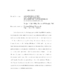## Asymptotics of the Yang-Mills Flow for Holomorphic Vector Bundles over Kahler ManifoldsIn this thesis we study the limiting properties of the Yang-Mills flow associated to a holomorphic vector bundle $E$ over an arbitrary K"{a}hler manifold $(X,omega )$. In particular we show that the flow is determined at infinity by the holomorphic structure of $E$. Namely, if we fix an integrable unitary reference connection $A_{0}$ defining the holomorphic structure, then the Yang-Mills flow with initial condition $A_{0}$, converges (away from an appropriately defined singular set) in the sense of the Uhlenbeck compactness theorem to a holomorphic vector bundle $E_{infty }$, which is isomorphic to the associated graded object of the Harder-Narasimhan-Seshadri filtration of $(E,A_{0})$. Moreover, $E_{infty }$ extends as a reflexive sheaf over the singular set as the double dual of the associated graded object. This is an extension of previous work in the cases of $1$ and $2$ complex dimensions and proves the general case of a conjecture of Bando and Siu. Chapter 1 is an introduction and a review of the background material. Chapter 2 gives the proof of several critical intermediate results, including the existence of an approximate critical hermitian structure. Chapter 3 concludes the proof of the main theorem.